# Understanding How PostgreSQL Executes a Query

Before going much further, you should understand the procedure that PostgreSQL follows whenever it executes a query on your behalf.

After the PostgreSQL server receives a query from the client application, the text of the query is handed to the parser. The parser scans through the query and checks it for syntax errors. If the query is syntactically correct, the parser will transform the query text into a parse tree. A parse tree is a data structure that represents the meaning of your query in a formal, unambiguous form.

Given the query

```SELECT customer_name, balance FROM customers WHERE balance > 0 ORDER BY balance
```

the parser might come up with a parse tree structured as shown in Figure 4.5.

Figure 4.5. A sample parse tree.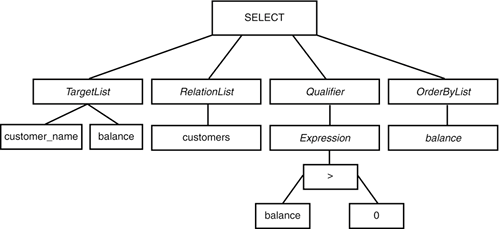After the parser has completed parsing the query, the parse tree is handed off to the planner/optimizer.

The planner is responsible for traversing the parse tree and finding all possible plans for executing the query. The plan might include a sequential scan through the entire table and index scans if useful indexes have been defined. If the query involves two or more tables, the planner can suggest a number of different methods for joining the tables. The execution plans are developed in terms of query operators. Each query operator transforms one or more input sets into an intermediate result set. The Seq Scan operator, for example, transforms an input set (the physical table) into a result set, filtering out any rows that don't meet the query constraints. The Sort operator produces a result set by reordering the input set according to one or more sort keys. I'll describe each of the query operators in more detail a little later. Figure 4.6 shows an example of a simple execution plan (it is a new example; it is not related to the parse tree in Figure 4.5).

Figure 4.6. A simple execution plan.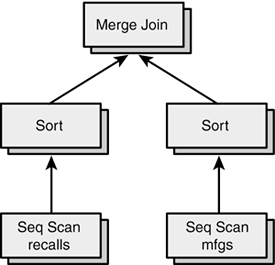You can see that complex queries are broken down into simple steps. The input set for a query operator at the bottom of the tree is usually a physical table. The input set for an upper-level operator is the result set of a lower-level operator.

When all possible execution plans have been generated, the optimizer searches for the least-expensive plan. Each plan is assigned an estimated execution cost. Cost estimates are measured in units of disk I/O. An operator that reads a single block of 8,192 bytes (8K) from the disk has a cost of one unit. CPU time is also measured in disk I/O units, but usually as a fraction. For example, the amount of CPU time required to process a single tuple is assumed to be 1/100th of a single disk I/O. You can adjust many of the cost estimates. Each query operator has a different cost estimate. For example, the cost of a sequential scan of an entire table is computed as the number of 8K blocks in the table, plus some CPU overhead.

After choosing the (apparently) least-expensive execution plan, the query executor starts at the beginning of the plan and asks the topmost operator to produce a result set. Each operator transforms its input set into a result setthe input set may come from another operator lower in the tree. When the topmost operator completes its transformation, the results are returned to the client application.

EXPLAIN

The EXPLAIN statement gives you some insight into how the PostgreSQL query planner/optimizer decides to execute a query.

First, you should know that the EXPLAIN statement can be used only to analyze SELECT, INSERT, DELETE, UPDATE, and DECLARE...CURSOR commands.

The syntax for the EXPLAIN command is

```EXPLAIN [ANALYZE][VERBOSE] query;
```

Let's start by looking at a simple example:

```perf=# EXPLAIN ANALYZE SELECT * FROM recalls;
NOTICE: QUERY PLAN:
Seq Scan on recalls (cost=0.00..9217.41 rows=39241 width=1917)
(actual time=69.35..3052.72 rows=39241 loops=1)
Total runtime: 3144.61 msec
```

The format of the execution plan can be a little mysterious at first. For each step in the execution plan, EXPLAIN prints the following information:

• The type of operation required.
• The estimated cost of execution.
• If you specified EXPLAIN ANALYZE, the actual cost of execution. If you omit the ANALYZE keyword, the query is planned but not executed, and the actual cost is not displayed.

In this example, PostgreSQL has decided to perform a sequential scan of the recalls table (Seq Scan on recalls). There are many operators that PostgreSQL can use to execute a query. I'll explain the operation type in more detail in a moment.

There are three data items in the cost estimate. The first set of numbers (cost=0.00..9217.41) is an estimate of how "expensive" this operation will be. "Expensive" is measured in terms of disk reads. Two numbers are given: The first number represents how quickly the first row in the result set can be returned by the operation; the second (which is usually the most important) represents how long the entire operation should take. The second data item in the cost estimate (rows=39241) shows how many rows PostgreSQL expects to return from this operation. The final data item (width=1917) is an estimate of the width, in bytes, of the average row in the result set.

If you include the ANALYZE keyword in the EXPLAIN command, PostgreSQL will execute the query and display the actual execution costs.

## Cost Estimates

I will remove the cost estimates from some of the EXPLAIN results in this chapter to make the plan a bit easier to read. Don't be confused by thisthe EXPLAIN command will always print cost estimates.

This was a simple example. PostgreSQL required only one step to execute this query (a seq uential scan on the entire table). Many queries require multiple steps and the EXPLAIN command will show you each of those steps. Let's look at a more complex example:

```perf=# EXPLAIN ANALYZE SELECT * FROM recalls ORDER BY yeartxt;
NOTICE: QUERY PLAN:

Sort (cost=145321.51..145321.51 rows=39241 width=1911)
(actual time=13014.92..13663.86 rows=39241 loops=1)

->Seq Scan on recalls (cost=0.00..9217.41 rows=39241 width=1917)
(actual time=68.99..3446.74 rows=39241 loops=1)
Total runtime: 16052.53 msec
```

This example shows a two-step query plan. In this case, the first step is actually listed at the end of the plan. When you read a query plan, it is important to remember that each step in the plan produces an intermediate result set. Each intermediate result set is fed into the next step of the plan.

Looking at this plan, PostgreSQL first produces an intermediate result set by performing a sequential scan (Seq Scan) on the entire recalls table. That step should take about 9,217 disk page reads, and the result set will have about 39,241 rows, averaging 1,917 bytes each. Notice that these estimates are identical to those produced in the first exampleand in both cases, you are executing a sequential scan on the entire table.

After the sequential scan has finished building its intermediate result set, it is fed into the next step in the plan. The final step in this particular plan is a sort operation, which is required to satisfy our ORDER BY clause. The sort operation reorders the result set produced by the sequential scan and returns the final result set to the client application.

 An ORDER BY clause does not require a Sort operation in all cases. The planner/optimizer may decide that it can use an index to order the result set.

The Sort operation expects a single operanda result set. The Seq Scan operation expects a single operanda table. Some operations require more than one operand. Here is a join between the recalls table and the mfgs table:

```perf=# EXPLAIN SELECT * FROM recalls, mfgs
perf-# WHERE recalls.mfgname = mfgs.mfgname;
NOTICE: QUERY PLAN:

Merge Join
-> Sort
-> Seq Scan on recalls
-> Sort
-> Seq Scan on mfgs
```

If you use your imagination, you will see that this query plan is actually a tree structure, as illustrated in Figure 4.7.

Figure 4.7. Execution plan viewed as a tree.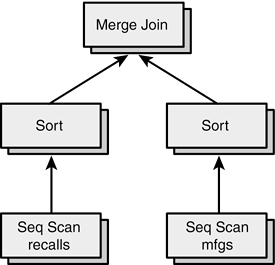When PostgreSQL executes this query plan, it starts at the top of the tree. The Merge Join operation requires two result sets for input, so PostgreSQL must move down one level in the tree; let's assume that you traverse the left child first. Each Sort operation requires a single result set for input, so again the query executor moves down one more level. At the bottom of the tree, the Seq Scan operation simply reads a row from a table and returns that row to its parent. After a Seq Scan operation has scanned the entire table, the left-hand Sort operation can complete. As soon as the left-hand Sort operation completes, the Merge Join operator will evaluate its right child. In this case, the right-hand child evaluates the same way as the left-hand child. When both Sort operations complete, the Merge Join operator will execute, producing the final result set.

So far, you've seen three query execution operators in the execution plans. PostgreSQL currently has 19 query operators. Let's look at each in more detail.

Seq Scan

The Seq Scan operator is the most basic query operator. Any single-table query can be carried out using the Seq Scan operator.

Seq Scan works by starting at the beginning of the table and scanning to the end of the table. For each row in the table, Seq Scan evaluates the query constraints (that is, the WHERE clause); if the constraints are satisfied, the required columns are added to the result set.

 The entire WHERE clause may not be evaluated for each row in the input set. PostgreSQL evaluates only the portions of the clause that apply to the given row (if any). For a single-table SELECT, the entire WHERE clause is evaluated. For a multi-table join, only the portion that applies to the given row is evaluated.

As you saw earlier in this chapter, a table can include dead (that is, deleted) rows and rows that may not be visible because they have not been committed. Seq Scan does not include dead rows in the result set, but it must read the dead rows, and that can be expensive in a heavily updated table.

The cost estimate for a Seq Scan operator gives you a hint about how the operator works:

```Seq Scan on recalls (cost=0.00..9217.41 rows=39241 width=1917)
```

The startup cost is always 0.00. This implies that the first row of a Seq Scan operator can be returned immediately and that Seq Scan does not read the entire table before returning the first row. If you open a cursor against a query that uses the Seq Scan operator (and no other operators), the first FETCH will return immediatelyyou won't have to wait for the entire result set to be materialized before you can FETCH the first row. Other operators (such as Sort) do read the entire input set before returning the first row.

The planner/optimizer chooses a Seq Scan if there are no indexes that can be used to satisfy the query. A Seq Scan is also used when the planner/optimizer decides that it would be less expensive (or just as expensive) to scan the entire table and then sort the result set to meet an ordering constraint (such as an iORDER BY clause).

Index Scan

An Index Scan operator works by traversing an index structure. If you specify a starting value for an indexed column (WHERE record_id >= 1000, for example), the Index Scan will begin at the appropriate value. If you specify an ending value (such as WHERE record_id < 2000), the Index Scan will complete as soon as it finds an index entry greater than the ending value.

The Index Scan operator has two advantages over the Seq Scan operator. First, a Seq Scan must read every row in the tableit can only remove rows from the result set by evaluating the WHERE clause for each row. Index Scan may not read every row if you provide starting and/or ending values. Second, a Seq Scan returns rows in table order, not in sorted order. Index Scan will return rows in index order.

Not all indexes are scannable. The B-Tree, R-Tree, and GiST index types can be scanned; a Hash index cannot.

The planner/optimizer uses an Index Scan operator when it can reduce the size of the result set by traversing a range of indexed values, or when it can avoid a sort because of the implicit ordering offered by an index.

Sort

The Sort operator imposes an ordering on the result set. PostgreSQL uses two different sort strategies: an in-memory sort and an on-disk sort. You can tune a PostgreSQL instance by adjusting the value of the sort_mem runtime parameter. If the size of the result set exceeds sort_mem, Sort will distribute the input set to a collection of sorted work files and then merge the work files back together again. If the result set will fit in sort_mem*1024 bytes, the sort is done in memory using the QSort algorithm.

A Sort operator never reduces the size of the result setit does not remove rows or columns.

Unlike Seq Scan and Index Scan, the Sort operator must process the entire input set before it can return the first row.

The Sort operator is used for many purposes. Obviously, a Sort can be used to satisfy an ORDER BY clause. Some query operators require their input sets to be ordered. For example, the Unique operator (we'll see that in a moment) eliminates rows by detecting duplicate values as it reads through a sorted input set. Sort will also be used for some join operations, group operations, and for some set operations (such as INTERSECT and UNION).

Unique

The Unique operator eliminates duplicate values from the input set. The input set must be ordered by the columns, and the columns must be unique. For example, the following command

```SELECT DISTINCT mfgname FROM recalls;
```

might produce this execution plan:

```Unique
-> Sort
-> Seq Scan on recalls
```

The Sort operation in this plan orders its input set by the mfgname column. Unique works by comparing the unique column(s) from each row to the previous row. If the values are the same, the duplicate is removed from the result set.

The Unique operator removes only rowsit does not remove columns and it does not change the ordering of the result set.

Unique can return the first row in the result set before it has finished processing the input set.

The planner/optimizer uses the Unique operator to satisfy a DISTINCT clause. Unique is also used to eliminate duplicates in a UNION.

LIMIT

The LIMIT operator is used to limit the size of a result set. PostgreSQL uses the LIMIT operator for both LIMIT and OFFSET processing. The LIMIT operator works by discarding the first x rows from its input set, returning the next y rows, and discarding the remainder. If the query includes an OFFSET clause, x represents the offset amount; otherwise, x is zero. If the query includes a LIMIT clause, y represents the LIMIT amount; otherwise, y is at least as large as the number of rows in the input set.

The ordering of the input set is not important to the LIMIT operator, but it is usually important to the overall query plan. For example, the query plan for this query

```perf=# EXPLAIN SELECT * FROM recalls LIMIT 5;
NOTICE: QUERY PLAN:

Limit (cost=0.00..0.10 rows=5 width=1917)
-> Seq Scan on recalls (cost=0.00..9217.41 rows=39241 width=1917)
```

shows that the LIMIT operator rejects all but the first five rows returned by the Seq Scan. On the other hand, this query

```perf=# EXPLAIN ANALYZE SELECT * FROM recalls ORDER BY yeartxt LIMIT 5;
NOTICE: QUERY PLAN:

Limit (cost=0.00..0.10 rows=5 width=1917)
->Sort (cost=145321.51..145321.51 rows=39241 width=1911)
->Seq Scan on recalls (cost=0.00..9217.41 rows=39241 width=1917)
```

shows that the LIMIT operator returns the first five rows from an ordered input set.

The LIMIT operator never removes columns from the result set, but it obviously removes rows.

The planner/optimizer uses a LIMIT operator if the query includes a LIMIT clause, an OFFSET clause, or both. If the query includes only a LIMIT clause, the LIMIT operator can return the first row before it processes the entire set.

Aggregate

The planner/optimizer produces an Aggregate operator whenever the query includes an aggregate function. The following functions are aggregate functions: AVG(), COUNT(), MAX(), MIN(), STDDEV(), SUM(), and VARIANCE().

Aggregate works by reading all the rows in the input set and computing the aggregate values. If the input set is not grouped, Aggregate produces a single result row. For example:

```movies=# EXPLAIN SELECT COUNT(*) FROM customers;
Aggregate (cost=22.50..22.50 rows=1 width=0)
-> Seq Scan on customers (cost=0.00..20.00 rows=1000 width=0)
```

If the input set is grouped, Aggregate produces one result row for each group:

```movies=# EXPLAIN
movies-# SELECT COUNT(*), EXTRACT( DECADE FROM birth_date )
movies-# FROM customers
movies-# GROUP BY EXTRACT( DECADE FROM birth_date );
NOTICE: QUERY PLAN:

Aggregate (cost=69.83..74.83 rows=100 width=4)
-> Group (cost=69.83..72.33 rows=1000 width=4)
-> Sort (cost=69.83..69.83 rows=1000 width=4)
-> Seq Scan on customers (cost=0.00..20.00 rows=1000 width=4)
```

Notice that the row estimate of an ungrouped aggregate is always 1; the row estimate of a group aggregate is 1/10th of the size of the input set.

Append

The Append operator is used to implement a UNION. An Append operator will have two or more input sets. Append works by returning all rows from the first input set, then all rows from the second input set, and so on until all rows from all input sets have been processed.

Here is a query plan that shows the Append operator:

```perf=# EXPLAIN
perf-# SELECT * FROM recalls WHERE mfgname = 'FORD'
perf-# UNION
perf=# SELECT * FROM recalls WHERE yeartxt = '1983';

Unique
->Sort
->Append
->Subquery Scan *SELECT* 1
->Seq Scan on recalls
->Subquery Scan *SELECT* 2
->Seq Scan on recalls
```

The cost estimate for an Append operator is simply the sum of cost estimates for all input sets. An Append operator can return its first row before processing all input rows.

The planner/optimizer uses an Append operator whenever it encounters a UNION clause. Append is also used when you select from a table involved in an inheritance hierarchy. In Chapter 3, "PostgreSQL SQL Syntax and Use," I defined three tables, as shown in Figure 4.8.

Figure 4.8. Inheritance hierarchy.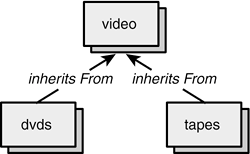The dvds table inherits from video, as does the tapes table. If you SELECT from dvds or video, PostgreSQL will respond with a simple query plan:

```movies=# EXPLAIN SELECT * FROM dvds;
Seq Scan on dvds (cost=0.00..20.00 rows=1000 width=122)

movies=# EXPLAIN SELECT * FROM tapes;
Seq Scan on tapes (cost=0.00..20.00 rows=1000 width=86)
```

Remember, because of the inheritance hierarchy, a dvd is a video and a tape is a video. If you SELECT from video, you would expect to see all dvds, all tapes, and all videos. The query plan reflects the inheritance hierarchy:

```movies=# EXPLAIN SELECT * FROM video;

Result(cost=0.00..60.00 rows=3000 width=86)
->Append(cost=0.00..60.00 rows=3000 width=86)
->Seq Scan on video (cost=0.00..20.00 rows=1000 width=86)
->Seq Scan on tapes video (cost=0.00..20.00 rows=1000 width=86)
->Seq Scan on dvds video (cost=0.00..20.00 rows=1000 width=86)
```

Look closely at the width clause in the preceding cost estimates. If you SELECT from the dvds table, the width estimate is 122 bytes per row. If you SELECT from the tapes table, the width estimate is 86 bytes per row. When you SELECT from video, all rows are expected to be 86 bytes long. Here are the commands used to create the tapes and dvds tables:

```movies=# CREATE TABLE tapes ( ) INHERITS( video );

movies=# CREATE TABLE dvds
movies-# (
movies(# region_id INTEGER,
movies(# audio_tracks VARCHAR[]
movies(# ) INHERITS ( video );
```

You can see that a row from the tapes table is identical to a row in the video tableyou would expect them to be the same size (86 bytes). A row in the dvds table contains a video plus a few extra columns, so you would expect a dvds row to be longer than a video row. When you SELECT from the video table, you want all videos. PostgreSQL discards any columns that are not inherited from the video table.

Result

The Result operator is used in three contexts.

First, a Result operator is used to execute a query that does not retrieve data from a table:

```movies=# EXPLAIN SELECT timeofday();
Result
```

In this form, the Result operator simply evaluates the given expression(s) and returns the results.

Result is also used to evaluate the parts of a WHERE clause that don't depend on data retrieved from a table. For example:

```movies=# EXPLAIN SELECT * FROM tapes WHERE 1 <> 1;
Result
->Seq Scan on tapes
```

This might seem like a silly query, but some client applications will generate a query of this form as an easy way to retrieve the metadata (that is, column definitions) for a table.

In this form, the Result operator first evaluates the constant part of the WHERE clause. If the expression evaluates to FALSE, no further processing is required and the Result operator completes. If the expression evaluates to TRUE, Result will return its input set.

The planner/optimizer also generates a Result operator if the top node in the query plan is an Append operator. This is a rather obscure rule that has no performance implications; it just happens to make the query planner and executor a bit simpler for the PostgreSQL developers to maintain.

Nested Loop

The Nested Loop operator is used to perform a join between two tables. A Nested Loop operator requires two input sets (given that a Nested Loop joins two tables, this makes perfect sense).

Nested Loop works by fetching each row from one of the input sets (called the outer table). For each row in the outer table, the other input (called the inner table) is searched for a row that meets the join qualifier.

Here is an example:

```perf=# EXPLAIN
perf-# SELECT * FROM customers, rentals
perf=# WHERE customers.customer_id = rentals.customer_id;

Nested Loop
-> Seq Scan on rentals
-> Index Scan using customer_id on customers
```

The outer table is always listed first in the query plan (in this case, rentals is the outer table). To execute this plan, the Nested Loop operator will read each row in the rentals table. For each rentals row, Nested Loop reads the corresponding customers row using an indexed lookup on the customer_id index.

 Actually, Nested Loop reads only those rows that meet the query constraints.

A Nested Loop operator can be used to perform inner joins, left outer joins, and unions.

Because Nested Loop does not process the entire inner table, it can't be used for other join types (full, right join, and so on).

Merge Join

The Merge Join operator also joins two tables. Like the Nested Loop operator, Merge Join requires two input sets: an outer table and an inner table. Each input set must be ordered by the join columns.

Let's look at the previous query, this time executed as a Merge Join:

```perf=# EXPLAIN
perf-# SELECT * FROM customers, rentals
perf=# WHERE customers.customer_id = rentals.customer_id;

Merge Join
-> Sort
-> Seq Scan on rentals
-> Index Scan using customer_id on customers
```

Merge Join starts reading the first row from each table (see Figure 4.9).

Figure 4.9. Merge JoinStep 1.If the join columns are equal (as in this case), Merge Join creates a new row containing the necessary columns from each input table and returns the new row. Merge Join then moves to the next row in the outer table and joins it with the corresponding row in the inner table (see Figure 4.10).

Figure 4.10. Merge JoinStep 2.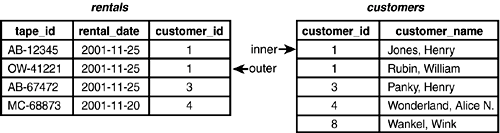Next, Merge Join reads the third row in the outer table (see Figure 4.11).

Figure 4.11. Merge JoinStep 3.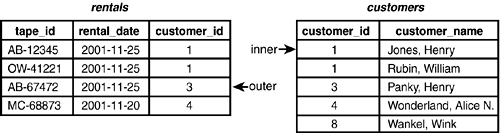Now Merge Join must advance the inner table twice before another result row can be created (see Figure 4.12).

Figure 4.12. Merge JoinStep 4.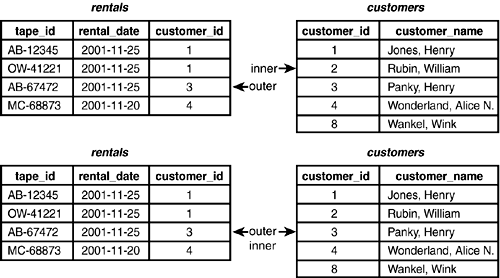After producing the result row for customer_id = 3, Merge Join moves to the last row in the outer table and then advances the inner table to a matching row (see Figure 4.13).

Figure 4.13. Merge JoinStep 5.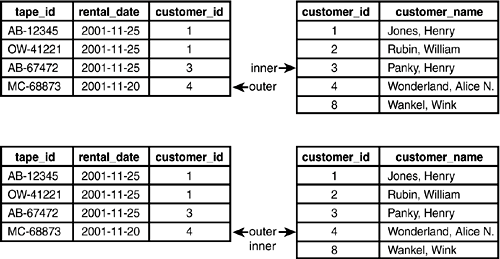Merge Join completes by producing the final result row (customer_id = 4).

You can see that Merge Join works by walking through two sorted tables and finding matchesthe trick is in keeping the pointers synchronized.

This example shows an inner join, but the Merge Join operator can be used for other join types by walking through the sorted input sets in different ways. Merge Join can do inner joins, outer joins, and unions.

Hash and Hash Join

The Hash and Hash Join operators work together. The Hash Join operator requires two input sets, again called the outer and inner tables. Here is a query plan that uses the Hash Join operator:

```movies=# EXPLAIN
movies-# SELECT * FROM customers, rentals
movies-# WHERE rentals.customer_id = customers.customer_id;

Hash Join
-> Seq Scan on customers
-> Hash
-> Seq Scan on rentals
```

Unlike other join operators, Hash Join does not require either input set to be ordered by the join column. Instead, the inner table is always a hash table, and the ordering of the outer table is not important.

The Hash Join operator starts by creating its inner table using the Hash operator. The Hash operator creates a temporary Hash index that covers the join column in the inner table.

Once the hash table (that is, the inner table) has been created, Hash Join reads each row in the outer table, hashes the join column (from the outer table), and searches the temporary Hash index for a matching value.

A Hash Join operator can be used to perform inner joins, left outer joins, and unions.

Group

The Group operator is used to satisfy a GROUP BY clause. A single input set is required by the Group operator, and it must be ordered by the grouping column(s).

Group can work in two distinct modes. If you are computing a grouped aggregate, Group will return each row in its input set, following each group with a NULL row to indicate the end of the group (the NULL row is for internal bookkeeping only, and it will not show up in the final result set). For example:

```movies=# EXPLAIN
movies-# SELECT COUNT(*), EXTRACT( DECADE FROM birth_date )
movies-# FROM customers
movies-# GROUP BY EXTRACT( DECADE FROM birth_date );
NOTICE: QUERY PLAN:

Aggregate (cost=69.83..74.83 rows=100 width=4)
-> Group (cost=69.83..72.33 rows=1000 width=4)
-> Sort (cost=69.83..69.83 rows=1000 width=4)
-> Seq Scan on customers (cost=0.00..20.00 rows=1000 width=4)
```

Notice that the row count in the Group operator's cost estimate is the same as the size of its input set.

If you are not computing a group aggregate, Group will return one row for each group in its input set. For example:

```movies=# EXPLAIN
movies-# SELECT EXTRACT( DECADE FROM birth_date ) FROM customers
movies-# GROUP BY EXTRACT( DECADE FROM birth_date );

Group (cost=69.83..69,83 rows=100 width=4)
-> Sort (cost=69.83..69.83 rows=1000 width=4)
-> Seq Scan on customers (cost=0.00..20.00 rows=1000 width=4)
```

In this case, the estimated row count is 1/10th of the Group operator's input set.

Subquery Scan and Subplan

A Subquery Scan operator is used to satisfy a UNION clause; Subplan is used for subselects. These operators scan through their input sets, adding each row to the result set. Each of these operators are used for internal bookkeeping purposes and really don't affect the overall query planyou can usually ignore them.

Just so you know when they are likely to be used, here are two sample query plans that show the Subquery Scan and Subplan operators:

```perf=# EXPLAIN
perf-# SELECT * FROM recalls WHERE mfgname = 'FORD'
perf-# UNION
perf=# SELECT * FROM recalls WHERE yeartxt = '1983';

Unique
->Sort
->Append
->Subquery Scan *SELECT* 1
->Seq Scan on recalls
->Subquery Scan *SELECT* 2
->Seq Scan on recalls

movies=# EXPLAIN
movies-# SELECT * FROM customers
movies-# WHERE customer_id IN
movies-# (
movies(# SELECT customer_id FROM rentals
movies(# );
NOTICE: QUERY PLAN:

Seq Scan on customers (cost=0.00..3.66 rows=2 width=47)
SubPlan
-> Seq Scan on rentals (cost=0.00..1.04 rows=4 width=4)
```

Tid Scan

The Tid Scan (tuple ID scan) operator is rarely used. A tuple is roughly equivalent to a row. Every tuple has an identifier that is unique within a tablethis is called the tuple ID. When you select a row, you can ask for the row's tuple ID:

```movies=# SELECT ctid, customer_id, customer_name FROM customers;
ctid | customer_id | customer_name
-------+-------------+----------------------
(0,1) | 3 | Panky, Henry
(0,2) | 1 | Jones, Henry
(0,3) | 4 | Wonderland, Alice N.
(0,4) | 2 | Rubin, William
(4 rows)
```

The "ctid" is a special column (similar to the OID) that is automatically a part of every row. A tuple ID is composed of a block number and a tuple number within the block. All the rows in the previous sample are stored in block 0 (the first block of the table file). The customers row for "Panky, Henry" is stored in tuple 3 of block 0.

After you know a row's tuple ID, you can request that row again by using its ID:

```movies=# SELECT customer_id, customer_name FROM customers
movies-# WHERE ctid = '(0,3)';
customer_id | customer_name
-------------+----------------------
4 | Wonderland, Alice N.
(1 row)
```

The tuple ID works like a bookmark. A tuple ID, however, is valid only within a single transaction. After the transaction completes, the tuple ID should not be used.

The Tid Scan operator is used whenever the planner/optimizer encounters a constraint of the form ctid = expression or expression = ctid.

The fastest possible way to retrieve a row is by its tuple ID. When you SELECT by tuple ID, the Tid Scan operator reads the block specified in the tuple ID and returns the requested tuple.

Materialize

The Materialize operator is used for some subselect operations. The planner/optimizer may decide that it is less expensive to materialize a subselect once than to repeat the work for each top-level row.

Materialize will also be used for some merge-join operations. In particular, if the inner input set of a Merge Join operator is not produced by a Seq Scan, an Index Scan, a Sort, or a Materialize operator, the planner/optimizer will insert a Materialize operator into the plan. The reasoning behind this rule is not obviousit has more to do with the capabilities of the other operators than with the performance or the structure of your data. The Merge Join operator is complex; one requirement of Merge Join is that the input sets must be ordered by the join columns. A second requirement is that the inner input set must be repositionable; that is, Merge Join needs to move backward and forward through the input set. Not all ordered operators can move backward and forward. If the inner input set is produced by an operator that is not repositionable, the planner/optimizer will insert a Materialize.

Setop (Intersect, Intersect All, Except, Except All)

There are four Setop operators: Setop Intersect, Setop Intersect All, Setop Except, and Setop Except All. These operators are produced only when the planner/optimizer encounters an INTERSECT, INTERSECT ALL, EXCEPT, or EXCEPT ALL clause, respectively.

All Setop operators require two input sets. The Setop operators work by first combining the input sets into a sorted list, and then groups of identical rows are identified. For each group, the Setop operator counts the number of rows contributed by each input set. Finally, each Setop operator uses the counts to determine how many rows to add to the result set.

I think this will be easier to understand by looking at an example. Here are two queries; the first selects all customers born in the 1960s:

```movies=# SELECT * FROM customers
movies-# WHERE EXTRACT( DECADE FROM birth_date ) = 196;
customer_id | customer_name | phone | birth_date | balance
-------------+----------------------+----------+------------+---------
3 | Panky, Henry | 555-1221 | 1968-01-21 | 0.00
4 | Wonderland, Alice N. | 555-1122 | 1969-03-05 | 3.00
```

The second selects all customers with a balance greater than 0:

```movies=# SELECT * FROM customers WHERE balance > 0;
customer_id | customer_name | phone | birth_date | balance
-------------+----------------------+----------+------------+---------
2 | Rubin, William | 555-2211 | 1972-07-10 | 15.00
4 | Wonderland, Alice N. | 555-1122 | 1969-03-05 | 3.00
```

Now, combine these two queries with an INTERSECT clause:

```movies=# EXPLAIN
movies-# SELECT * FROM customers
movies-# WHERE EXTRACT( DECADE FROM birth_date ) = 196
movies-# INTERSECT
movies-# SELECT * FROM customers WHERE balance > 0;
SetOp Intersect
-> Sort
-> Append
-> Subquery Scan *SELECT* 1
-> Seq Scan on customers
-> Subquery Scan *SELECT* 2
-> Seq Scan on customers
```

The query executor starts by executing the two subqueries and then combining the results into a sorted list. An extra column is added that indicates which input set contributed each row:

``` customer_id | customer_name | birth_date | balance | input set
-------------+----------------------+------------+---------+----------
2 | Rubin, William | 1972-07-10 | 15.00 | inner
3 | Panky, Henry | 1968-01-21 | 0.00 | outer
4 | Wonderland, Alice N. | 1969-03-05 | 3.00 | outer
4 | Wonderland, Alice N. | 1969-03-05 | 3.00 | inner
```

The SetOp operator finds groups of duplicate rows (ignoring the input set pseudo-column). For each group, SetOp counts the number of rows contributed by each input set. The number of rows contributed by the outer set is called count(outer). The number of rows contributed by the inner result set is called count(inner).

Here is how the sample looks after counting each group:

``` customer_id | customer_name | birth_date | balance | input set
-------------+----------------------+------------+---------+----------
2 | Rubin, William | 1972-07-10 | 15.00 | inner
count(outer) = 0
count(inner) = 1
3 | Panky, Henry | 1968-01-21 | 0.00 | outer
count(outer) = 1
count(inner) = 0
4 | Wonderland, Alice N. | 1969-03-05 | 3.00 | outer
4 | Wonderland, Alice N. | 1969-03-05 | 3.00 | inner
count(outer) = 1
count(inner) = 1
```

The first group contains a single row, contributed by the inner input set. The second group contains a single row, contributed by the outer input set. The final group contains two rows, one contributed by each input set.

When SetOp reaches the end of a group of duplicate rows, it determines how many copies to write into the result set according to the following rules:

• INTERSECT If count(outer) > 0 and count(inner) > 0, write one copy of the row to the result set; otherwise, the row is not included in the result set.
• INTERSECT ALL If count(outer) > 0 and count(inner) > 0, write n copies of the row to the result set; where n is the greater count(outer) and count(inner).
• EXCEPT If count(outer) > 0 and count(inner) = 0, write one copy of the row to the result set.
• EXCEPT ALL If count(inner) >= count(outer), write n copies of the row to the result set; where n is count(outer) - count(inner).PostgreSQL(c) The comprehensive guide to building, programming, and administering PostgreSQL databases
ISBN: 735712573
EAN: N/A
Year: 2004
Pages: 261